# Chapter 7 Chapter 7: Getting Started with Generalized Linear Models

• Response variables in linear model-based analyses have several common features including that:
• We assume they are continuous variables that can take negative and positive values and can be fractins
• We also assume that these data are bounded (but in practice, sometimes they are not)
• The models assume normally distributed residuals
• The model also assumes a constant mean-variance relationship
• Often there are response variables in which the normality assumption is violated
• Examples of data that violate assumptions:
• Integer valued data that is bounded and cannot be fractioned or negative (i.e. the number of people)
• Binary data (i.e. presence/absence data)
• Historically for these types of data, transformations are employed such as log10 transformations for counts or arcsin(sqrt()) transformations for proportion data
• This is where the generalized linear model (GLM) may be utilized

### 7.0.1 Counts and Proportions and the GLM

• Count data:
• Are bounded by 0 and infinity
• Violate the normality assumption
• Do not have a constant mean-variance relationship
• Example:
• How the number of offspring a female may produce over her lifetime relates to body size
• How a rate of occurence of events (births) depends on other variables (body size)
• Proportion data - data are often binary
• flowering data
• animal death
• species sex ratios
• These occurences are often related to 1+ explanatory variables
• These data are binomial

### 7.0.2 Key terms of GLM models

• Family
• The probability distribution that is assumed to describe the response variable
• In other words, it’s a mathematical statement of how likely events are
• Poisson and binomial distributions are examples of families
• Linear predictor
• This is an equation that describes how the different predictor variables (explanatory variables) affect the expected value of the response variable
• Describes the mathematical relationship between the expected value of the response variable and the linear predictor, linking, the two aspects of the GLM
• glm() will be used to create generalized linear models

## 7.1 Counts and rates - Poisson GLMs

### 7.1.1 Counting Sheep - the data and question

• In this dataset, the response variable is a count variable
• We aim to understand how the rate of occurence of events depends on 1 or more explanatory variables
• Dataset backstory:
• It’s about a specific population of sheep
• These sheep are on an island off the west coast of Scotland
• They are unmanaged/feral population of Soay sheep
• There has been a lot of scientific interest on this population to study how the population is evolving
• Several studies have been done on female (ewe) fitness
• One way to measure fitness is to count the total number of offspring born to a female during her life - ‘lifetime reproductive success’
• response variable = counts of offspring, which are poorly approximated by a normal distribution
• question: Does lifetime reproductive success increase with ewe bodymass?
• Do larger ewes produce more offspring?
• If so, then is there a heritable difference in body mass - is there selection pressure on this trait?
install.packages("ggplot2",  repos = "https://cran.us.r-project.org")
install.packages("dplyr",  repos = "https://cran.us.r-project.org")
install.packages("ggfortify",  repos = "https://cran.us.r-project.org")
library(ggplot2)
library(dplyr)
library(ggfortify)
soay <- read.csv("/Users/peteapicella/Documents/R_tutorials/GSwR/SoaySheepFitness.csv", header = TRUE)
str(soay)
## 'data.frame':    50 obs. of  2 variables:
##  $fitness : int 4 3 2 14 5 2 2 5 8 4 ... ##$ body.size: num  6.37 7.18 6.16 8.6 7.33 ...

Visualize the data:

ggplot(soay, aes(x = body.size, y = fitness)) +
geom_point() +
geom_smooth(method = "lm", se = FALSE) + #applies linear regression (blue) line
geom_smooth(span = 1,                    #determines how wiggly the curve is
colour = "red", se = FALSE) + #applies a non-linear and more  flexible statistical model
xlab("Body mass (kg)") + ylab("Lifetime fitness")
## geom_smooth() using formula 'y ~ x'
## geom_smooth() using method = 'loess' and formula 'y ~ x'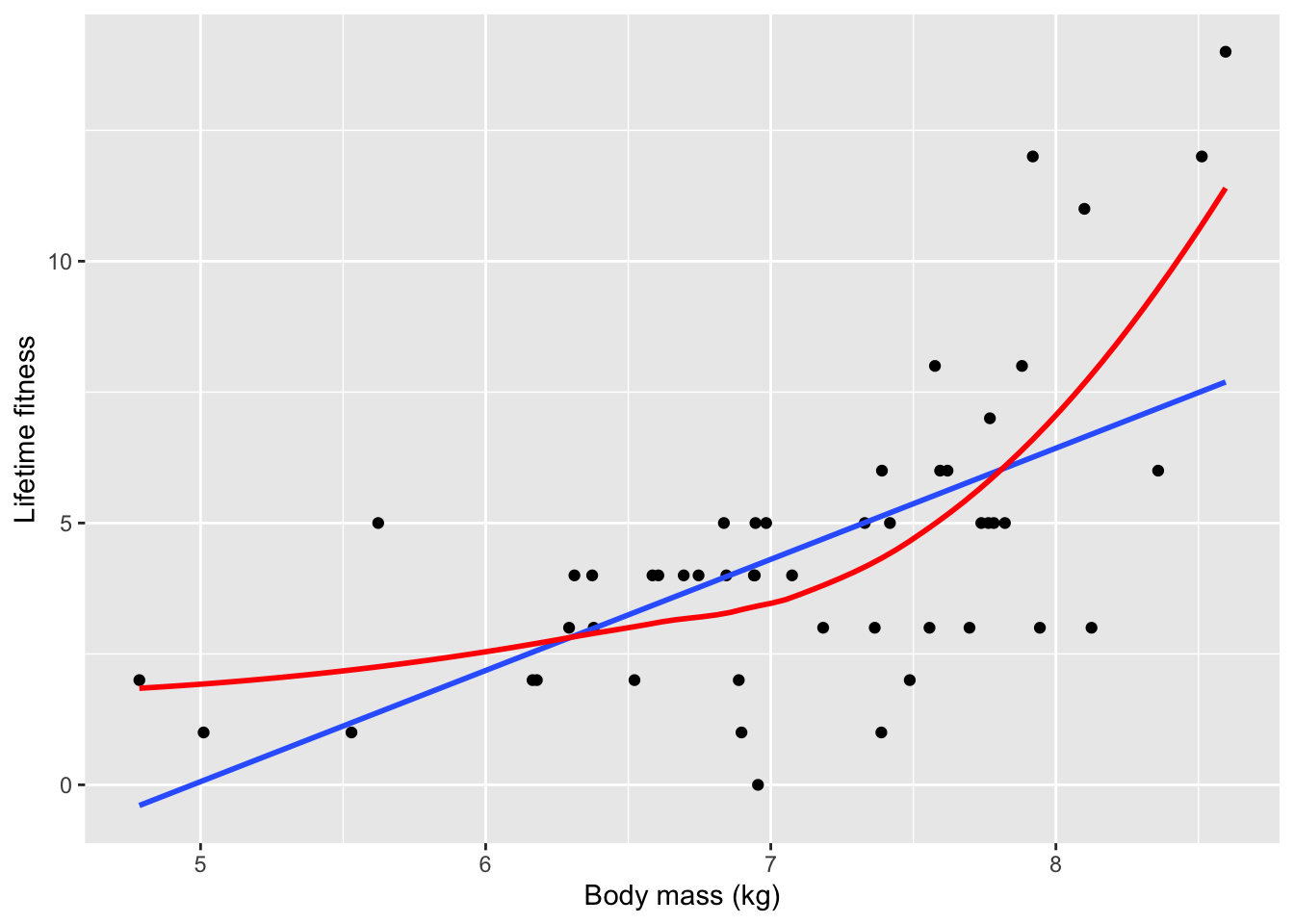• Based on the loose fitting models, there is a strong positive relationship between fitness and body size in which larger ewes have more offspring over time
• Larger ewes have more resources to allocate to reproduction
• The red line clearly captures the relationship more effectively than the blue one
• The upward trending of the red line is an issue of non-linearity
• There are also subtler issues with the data
• To really understand these problems, the authors want to analyze the data the incorrect way and then right the wrongs with the correct analysis after

## 7.2 Doing it wrong

Create an inappropriate model using the linear model approach:

soay.mod <- lm(fitness ~ body.size, data = soay)
autoplot(soay.mod, smooth.colour = NA)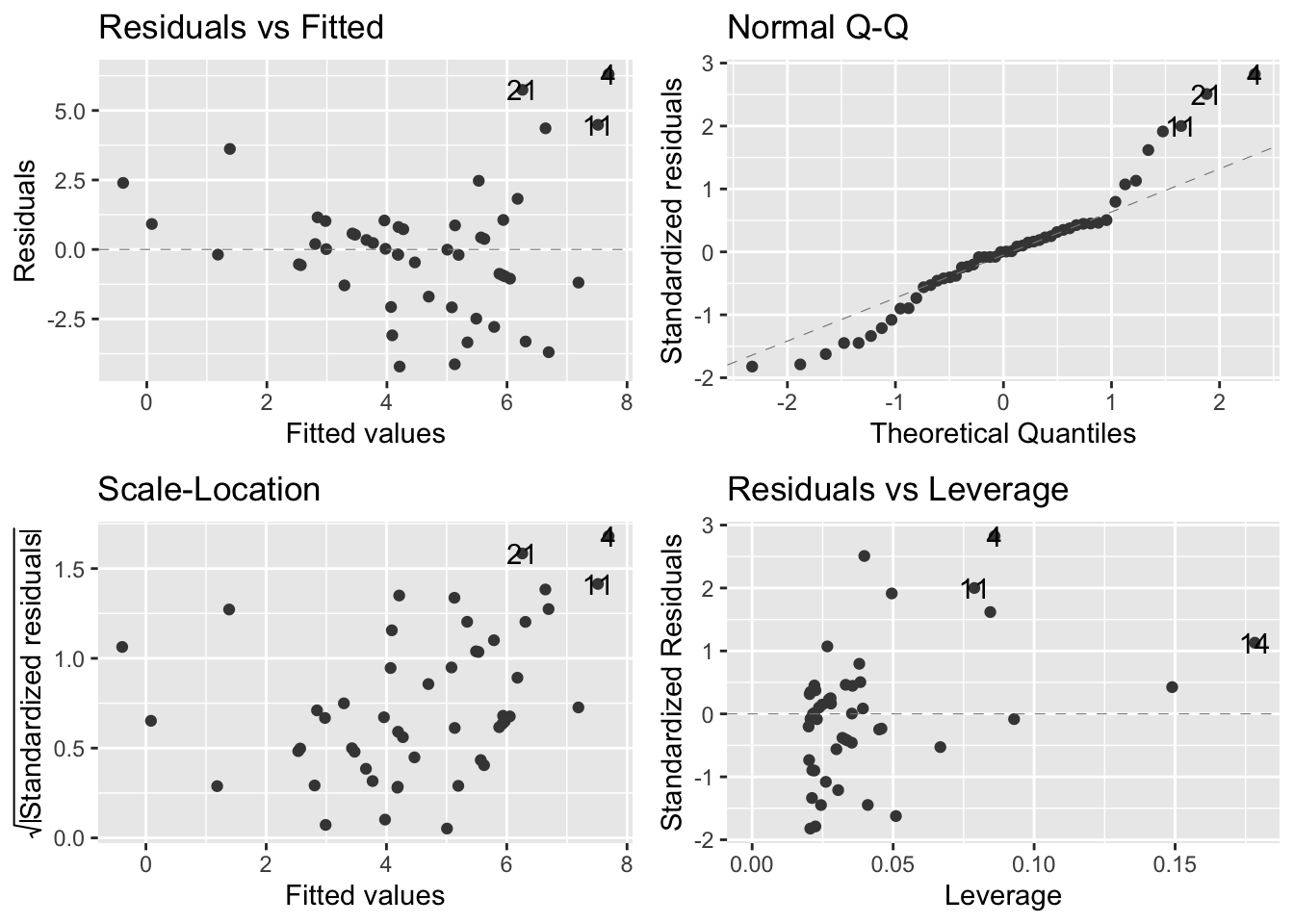• Almost all of our assumptions are violated

### 7.2.1 Doing it wrong: diagnosing the problems

• Residuals vs fitted values (upper left):
• Suggests that the systematic part of the model is inappropriate because there is a pattern of the datapoints
• In fact, the U-shape of the data indicate that the straight-line model fails to account for the curvature in the relationship between our two variables
• Fitness is underestimated at small body sizes
• Fitness is overestimated at larger body sizes
• Normal Q-Q plot (upper right):
• Most of the points should be on the dashed diagnonal line and they are not
• Many of the points in positive territory are above the line
• Many of the points in negative territory are below the line
• We see this pattern because the distribution of residuals is not symmetrical
• In this case, it is skewed to the right

Visualize rightward skew through a histogram:

ggplot(soay, aes(x=body.size))+ geom_histogram(bins=20)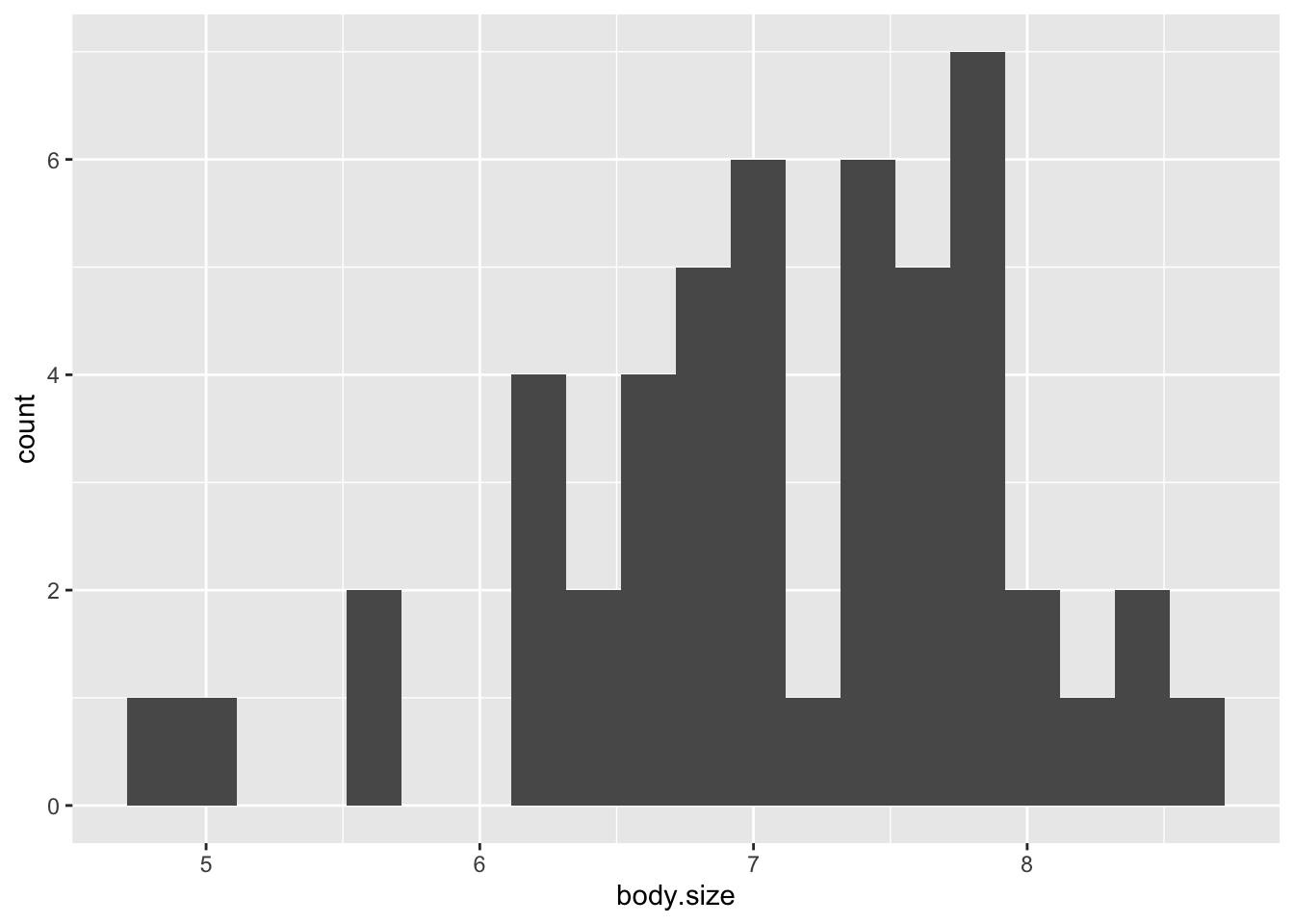• Scale location plot (bottom left):
• There is a clear pattern that shows a positive relationship between the absolute size of the residuals and the fitted values
• This reflects the way the fitness values are scattered around the red line: there is more vertical spread in the data at larger fitness values
• Based on this, we can say that there is a positive mean-variance relationship in the data
• In other words, large predicted fitness values are associated with more variation in the residuals
• This is typical of count data
• Residuals-leverage plot (bottom right):
• This plot is not too bad
• There are no extreme standardized residual values
• No obvious outliers
• None of the observations are having an outsized effect on the model

Overall, the normal linear regression is not doing a good job of describing the data

### 7.2.2 The Poisson distribution - a solution

• The normality assumption built into the linear regression model is not appropriate for these data - Properties of a normal distribution:
• Concerns continuous variables (can assume fractional values)
• Count data are discete (i.e. a ewe can produce 0,1,2, etc. lambs)
• Normal distribution allows for negative values
• Count data must be positive (i.e. ewe cannot have -2 offspring)
• Symmetry.
• Count data often are asymmetrical
• Poisson distribution is a good starting point for the analysis of certain forms of count data

A visual for understanding the Poisson distribution: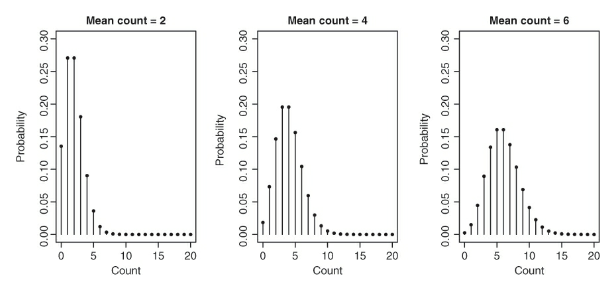• The above figure displays three Poisson distributions and each has a different mean
• The x-axis has a range of different possible values and the y axis has the probability of each value
• Reasons why the poisson distribution is a good model for the Soay data:
• Only discete counts are possible
• Data are bounded at 0
• Very large counts are possible and in the scope of the model; however, their occurence is unlikely
• Variance of the distribution increases as the mean of distribution is increased
• This corresponds to the widening of the base the distribution with a higher mean
• The poisson distribution is best equipped for unbounded count data
• What unbounded here refers to is just that there is no uppper limit to the values that the count variable may take
• There are obvious biological constraints, but we don’t actually know the limit

## 7.3 Doing it right - the Poisson GLM

### 7.3.1 Anatomy of a GLM

• GLM is comprised of three key terms: family, linear predictor, the link function

• family:

• This is the error aspect of the GLM
• Determines what kind of distribution is used to describe the response variable. Options:
• Poisson distribution
• Binomial distribution
• Gamma distribution (positive valued, continuous variables)
• Other exotic versions
• Each option is appropriate for specific types of data
• linear predictor:

• Every time a model is built with the lm() function, some data and an R formula must be supplied to define the model
• The R formula defines the linear predictor
• Soay example:
• inappropriate regression with model: lm(fitness ~ body.size, data = soay)
• This tells R to build a model for the predicted fitness given an intercept and body size slope term: Predicted fitness = Intercept + Slope x Body size
• The linear predictor is ‘Intercept + Slope x Body size’
• so it’s basically just the model
• Coefficients shown by summary(soay.mod) are just the intercepts and slopes of th linear predictor

• A model that cannot make impossible predictions is preferred
• You can plug numbers into the formul that will produce illogical outputs such that:
• A negative number of offspring in this sheep example
• With the GLM, instead of trying to model the predicted values of the response variable directly, we model a mathematical transformation of the prediction
• The transformation is the link function
• Using a Poisson GLM to model the fitness vs body mass relationship, the model for the predicted fitness would look like this:
• Log[Predicted fitness] = Intercept + Slope x Body size
• The link function in a standard Poisson GLM is always natural log
• Instead of the linear predictor describing fitness directly, it relates the natural logarithm of predicted fitness to body size
• This must be positive, but the log transformed value can take any value
• Solve for predicted fitness to get:
• Predicted fitness = e^{Intercept + Slope x Body size}
• A Poisson GLM for the Soays sheep implies an exponential relationship between fitness and body mass

• linear model does not mean a linear relationship
• The link function allows for the estimation of the paramenteres of a linear predictor that is apporpriate for the data

• This is accomplished by transforming the response ‘scale’ to the scale of the linear predictor, which in this case a natural log scale, which is defined by the link function
• This is can be visualized in the following figure: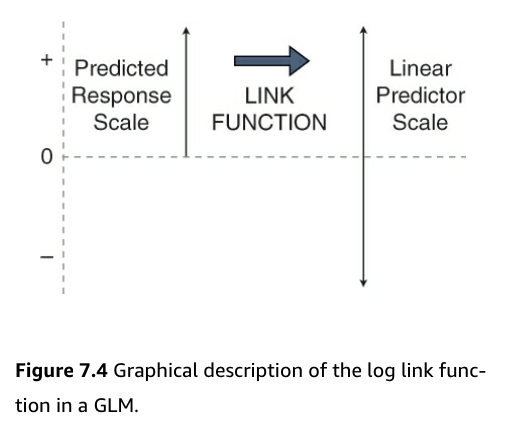• Figure:
• Count data is bounded by 0 and positive infinity
• Predicted values must be greater than 0 to be valid for count data
• But, to do effective statistics, we need to operate on a scale that is unbounded
• Using the link function accomplishes this
• It moves us from the positive numbers (predicted average counts) scale to the whole number line (the linear predictor) scale

### 7.3.2 Doing it right - actually fitting the model

Build the GLM:

soay.glm <- glm(fitness ~ body.size, data = soay,
family = poisson(
link = log)) #this line is unnecessary because the log link function is the default for poisson glm 

### 7.3.3 Doing it right - the diagnostics

autoplot(soay.glm, smooth.colour = NA)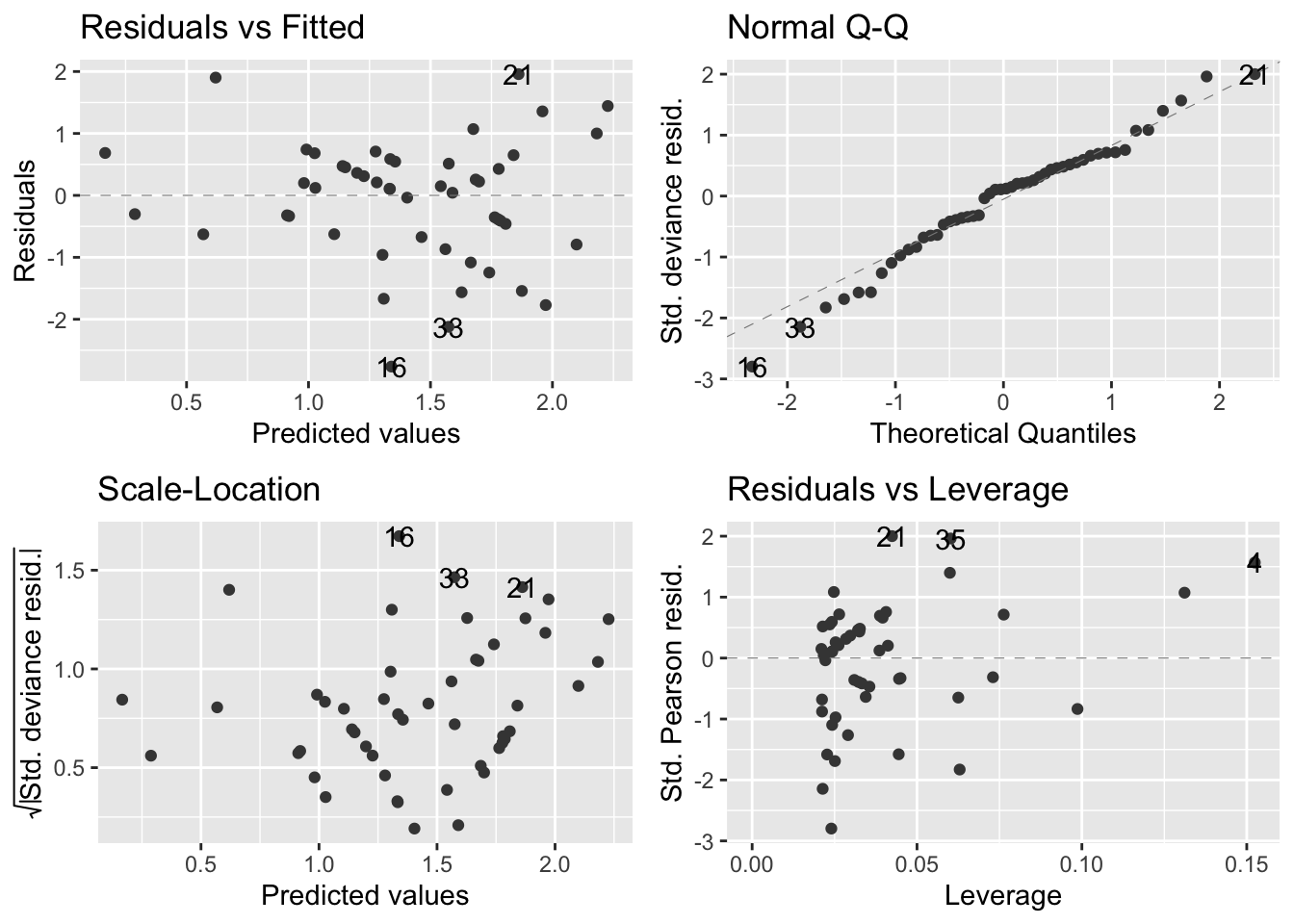• Overall, the diagnostics look much better
• Residuals vs fitted values (upper left):
• The systematic aspect of the model is fairly appropriate
• There is no clear pattern in the relationship apart from a very slight upward trend
• Not enough to be concerned with though
• Normal Q-Q plot (upper right):
• There is some departure from the dashed diagnonal line, but a perfect plot is not expected
• Confirms that the distributional assumptions are okay
• Scale location plot (bottom left):
• There is a slight positve relationship between the size of the residuals and the fitted values, but not too much of a concern
• Residuals-leverage plot (bottom right):
• Shows no evidence that there are outliers or points having an outsized effect on the model
• Keep in mind that when R does diagnostics for a GLM, it uses standardized deviance residuals
• This is a transformed version of raw residuals that make transformed residuals normally distributed if the GLM family that is being used is appropriate
• This means that if the chosen family is appropriate for the data, then the diagnostics should show that the residuals are normally distributed
• And the diagnostic plots can be evaluated in the same way as for a linear model because the tests are doing all the same jobs

### 7.3.4 Doing it right - anova() and summary()

• Thus far it looks that fitnesss is positively related to body mass
• The next step is to test hypotheses

Create Analysis of Deviance table for the GLM:

anova(soay.glm)
## Analysis of Deviance Table
##
##
## Response: fitness
##
## Terms added sequentially (first to last)
##
##
##           Df Deviance Resid. Df Resid. Dev
## NULL                         49     85.081
## body.size  1   37.041        48     48.040
• “Deviance” here is closely related to the idea of likelihood, which is a general tool for doing statistics
• Short explanation of likelihood: provides us with a measure of how probable the data would be if they had really been produced by that model
• Using likelihood, you can find a set of best-fitting model coefficients by picking values that maximize likelihood
• Also, sum of squares and mean squares allow for the comparison of different models when normality is assumed
• Likelihood (and deviance) do the same thing for GLMs
• no p values in this table
• Total deviance for NULL (fitness) is 85.081 and body.size explains 37.041 units of the deviance - So bodysize accounts for almost half of the deviance. This is very large.
• There is no p value because R wants the type of test to calculate it to be specified
• p values in the GLM involve a $$\chi$$$$^{2}$$ distribution rather than an F-distribution (this does not mean that a $$\chi$$$$^{2}$$ test is to be performed)

Specifiy the type of test:

anova(soay.glm, test = "Chisq")
## Analysis of Deviance Table
##
##
## Response: fitness
##
## Terms added sequentially (first to last)
##
##
##           Df Deviance Resid. Df Resid. Dev  Pr(>Chi)
## NULL                         49     85.081
## body.size  1   37.041        48     48.040 1.157e-09 ***
## ---
## Signif. codes:  0 '***' 0.001 '**' 0.01 '*' 0.05 '.' 0.1 ' ' 1
• The test statistic has a value of 37.041
• The corresponding p value is very small
• This is unsurprising given the strong relationship visualized in the scatterplot
• This is a likelihood ratio test
• This means that fitness does vary positively with body size and there is selection pressure on higher body mass

Find out more about the model:

summary(soay.glm)
##
## Call:
## glm(formula = fitness ~ body.size, family = poisson(link = log),
##     data = soay)
##
## Deviance Residuals:
##     Min       1Q   Median       3Q      Max
## -2.7634  -0.6275   0.1142   0.5370   1.9578
##
## Coefficients:
##             Estimate Std. Error z value Pr(>|z|)
## (Intercept) -2.42203    0.69432  -3.488 0.000486 ***
## body.size    0.54087    0.09316   5.806 6.41e-09 ***
## ---
## Signif. codes:  0 '***' 0.001 '**' 0.01 '*' 0.05 '.' 0.1 ' ' 1
##
## (Dispersion parameter for poisson family taken to be 1)
##
##     Null deviance: 85.081  on 49  degrees of freedom
## Residual deviance: 48.040  on 48  degrees of freedom
## AIC: 210.85
##
## Number of Fisher Scoring iterations: 4
• Table output:
• First chunk is self explanatory
• Second chunk - “useless” summary of specially scaled (deviance) residuals
• Third chunk - coefficients
• model is a line so there are only two coefficients - the intercept and a slope
• Each coefficient has a standard error to tell us how precise it is and a z-value to help us determine if the estimate is significantly different from 0 and the associated p value
• Fourth chunk - dispersion parameter - more on this later
• Fifth chunk - summaries of the null deviance, residual deviance, and their dfs
• null deviance - measure of all the ‘variation’ in the data
• residual deviance - measure of what is left over after fitting the model
• The bigger the difference in these two values, the more variation is explained by the model
• AIC = Akaike information criterion (not analyzed in this text)
• Number of Fisher Scoring iterations - not important
• Upon looking at the original scatterplot that helped visualize the data and this summary table, it might be confusing to see that the intercept has a negative value
• But remember that the link function is being used to predict the natural logarithm of lifetime fitness, not actual fitness
• We will revisit the overdispersion concept later in the chapter

### 7.3.5 Making a beautiful graph

• Need to generate a set of new x values

Generate new x values:

min.size = min(soay$body.size) max.size = max(soay$body.size)

new.x = expand.grid(body.size =
seq(min.size, max.size, #the new x values will be between these two parameters
length = 1000)) #R generates 1000 values between 
• Now the predict() function can be used:
• predict() is given three arguments: the GLM model, a value for newdata, and a request for standard errors
• Side note: predict() for glm does not have a confidence interval argument, so they have to be manually rendered into the dataframe
new.y <- predict(soay.glm,
newdata = new.x, #instructs R to use the new x values
se.fit = TRUE) #provides standard errors.predict() for glm() doesn't have an interval = confidence argument
new.y = data.frame(new.y) #converts new.y into a dataframe
head(new.y)
##         fit    se.fit residual.scale
## 1 0.1661991 0.2541777              1
## 2 0.1682619 0.2538348              1
## 3 0.1703247 0.2534919              1
## 4 0.1723874 0.2531491              1
## 5 0.1744502 0.2528063              1
## 6 0.1765130 0.2524635              1

Combine new x values with new y values:

addThese = data.frame(new.x, new.y)

head(addThese)
##   body.size   fitness    se.fit residual.scale
## 1  4.785300 0.1661991 0.2541777              1
## 2  4.789114 0.1682619 0.2538348              1
## 3  4.792928 0.1703247 0.2534919              1
## 4  4.796741 0.1723874 0.2531491              1
## 5  4.800555 0.1744502 0.2528063              1
## 6  4.804369 0.1765130 0.2524635              1

Calculate and include the confidence intervals in the dataframe:

addThese = mutate(addThese,
lwr = fitness -1.96 * se.fit, #this and the line below add the lower and upper bounds of the 95% CI
upr = fitness +1.96 * se.fit)
head(addThese)
##   body.size   fitness    se.fit residual.scale        lwr       upr
## 1  4.785300 0.1661991 0.2541777              1 -0.3319891 0.6643874
## 2  4.789114 0.1682619 0.2538348              1 -0.3292543 0.6657781
## 3  4.792928 0.1703247 0.2534919              1 -0.3265195 0.6671689
## 4  4.796741 0.1723874 0.2531491              1 -0.3237848 0.6685597
## 5  4.800555 0.1744502 0.2528063              1 -0.3210501 0.6699506
## 6  4.804369 0.1765130 0.2524635              1 -0.3183156 0.6713415

Visualize the data with our new model + predicted values:

ggplot(soay, aes(x = body.size, y = fitness)) +
geom_point(size = 3, alpha = 0.5) +
aes(ymin = lwr, ymax = upr), stat = 'identity') +
theme_bw()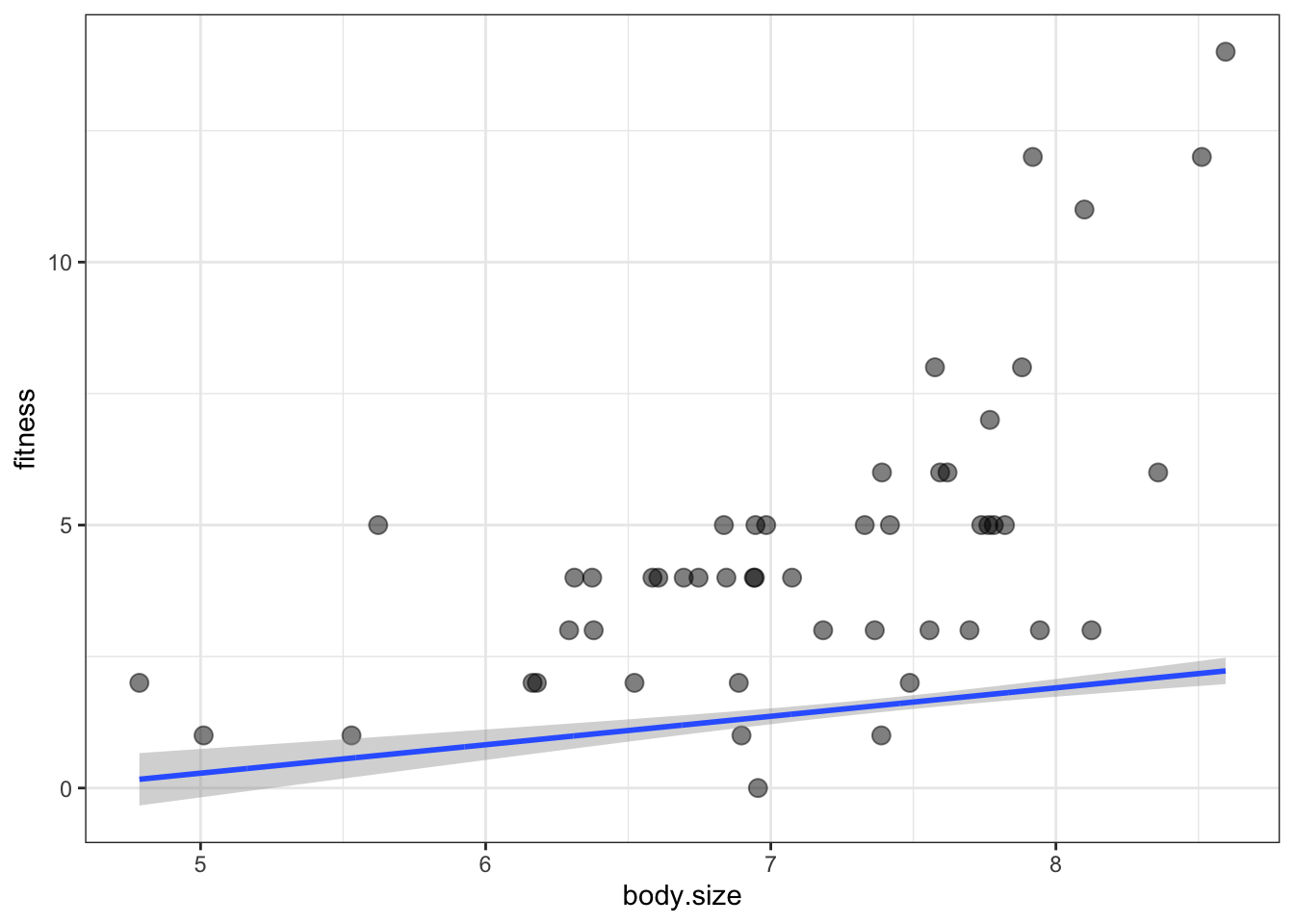it didn’t work:

• What happened is that the default scale for predict() is just the scale of the link function
• The link function uses a logarithmic scale
• This means that the predictions are the log of the expected fitness, but we want to plot the actual fitness values
• To fix this, the inverse log must be applied to any y-axis variables in addThese
• To inverse log, we just have to exponentiate them

Recreate the y values:

min.size = min(soay$body.size) max.size = max(soay$body.size)

new.x = expand.grid(body.size = seq(min.size, max.size, length = 1000))

new.y <- predict(soay.glm,
newdata = new.x,
se.fit = TRUE)
new.y = data.frame(new.y)
head(addThese)
##   body.size       fit    se.fit residual.scale
## 1  4.785300 0.1661991 0.2541777              1
## 2  4.789114 0.1682619 0.2538348              1
## 3  4.792928 0.1703247 0.2534919              1
## 4  4.796741 0.1723874 0.2531491              1
## 5  4.800555 0.1744502 0.2528063              1
## 6  4.804369 0.1765130 0.2524635              1

Exponentiate the y values:

addThese = mutate(addThese,
fitness = exp(fit),
lwr = exp(fit - 1.96 *se.fit),
upr = exp(fit - 1.96*se.fit))
head(addThese)
##   body.size       fit    se.fit residual.scale  fitness       lwr       upr
## 1  4.785300 0.1661991 0.2541777              1 1.180808 0.7174951 0.7174951
## 2  4.789114 0.1682619 0.2538348              1 1.183246 0.7194600 0.7194600
## 3  4.792928 0.1703247 0.2534919              1 1.185690 0.7214303 0.7214303
## 4  4.796741 0.1723874 0.2531491              1 1.188138 0.7234059 0.7234059
## 5  4.800555 0.1744502 0.2528063              1 1.190591 0.7253869 0.7253869
## 6  4.804369 0.1765130 0.2524635              1 1.193050 0.7273732 0.7273732

Revisulize the data:

ggplot(soay, aes(x = body.size, y = fitness)) +
geom_point(size = 3, alpha = 0.5) +
aes(ymin = lwr, ymax = upr), stat = 'identity') +
theme_bw()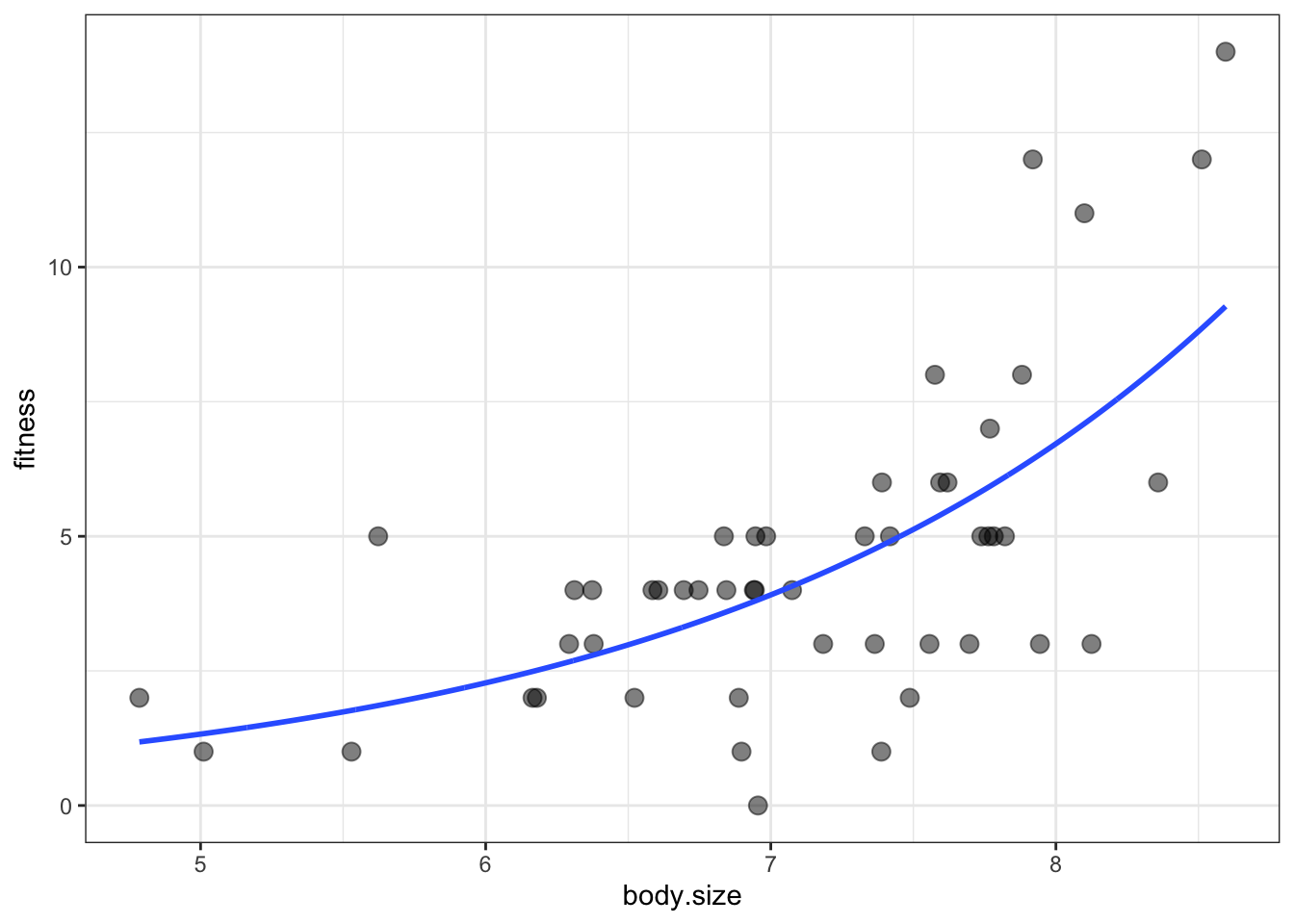## 7.4 When a Poisson GLM isn’t good for counts

• The previous data set was the best case scenario for a GLM and the simplest use case
• Now we will work with more realistic data

### 7.4.1 Overdispersion

• Overdispersion means ‘extra variation’
• GLMs make strong assumptions about the nature of variability in the data
• We have seen how the variance increases with the mean
• For a Poisson distribution, the variance is equal to the mean
• This assumption can only be true if you can account for every source of variation in the data (big assumption esp in biology)
• But Biology creates variation that cannot be measured or accounted for
• This is the source of the overdisperson problem
• From non-independence in the data
• Non-independence refers to the idea that some elements in the data are more similiar to one another than they are to other things
• i.e. cannabis plants in experiment are more similiar to each other than a random plant
• overdispersion sounds like BS but it can really mess up statistical output if it is ignored (i.e. p-values) and end up with false positives
• What to do about it?
• First: detect it.
summary(soay.glm)
##
## Call:
## glm(formula = fitness ~ body.size, family = poisson(link = log),
##     data = soay)
##
## Deviance Residuals:
##     Min       1Q   Median       3Q      Max
## -2.7634  -0.6275   0.1142   0.5370   1.9578
##
## Coefficients:
##             Estimate Std. Error z value Pr(>|z|)
## (Intercept) -2.42203    0.69432  -3.488 0.000486 ***
## body.size    0.54087    0.09316   5.806 6.41e-09 ***
## ---
## Signif. codes:  0 '***' 0.001 '**' 0.01 '*' 0.05 '.' 0.1 ' ' 1
##
## (Dispersion parameter for poisson family taken to be 1)
##
##     Null deviance: 85.081  on 49  degrees of freedom
## Residual deviance: 48.040  on 48  degrees of freedom
## AIC: 210.85
##
## Number of Fisher Scoring iterations: 4
• If a GLM is working appropriately and there is no overdispersion, then residual deviance (48.040) and its degrees of freedom (48) should be equal
• Perform $$\frac{residual~deviance}{residual~degrees~of~freedom}$$
• Then the output is a ‘dispersion index (DI)’ which should be approximately 1
• If much bigger than 1, then the data are overdispersed
• As a rule of thumb, if $$DI \ge 2$$
• If it is much less than 1, then the data are underdispersed (this is rare)
• Fortunately, the soay data have normal dispersion levels
• If data are overdispersed, then you can model the data differently
• A simple fix is to change the family in glm() from family = poisson to family = quasipoisson
• a quasi model works just like the glm but it also estimates the dispersion index in a much more clever way than we did
• Once this index value is known, then R can adjust the p-values accordingly
• Can also switch to a negative binomial family
• A negative binomial distribution is thought of as being more flexible than a Poisson distribution
• The variance increases with the mean, but in a less constrained way. The variance does not have to equal the mean
soay.glm2 <- glm(fitness ~ body.size, data = soay,
family = quasipoisson(
summary(soay.glm2)
##
## Call:
## glm(formula = fitness ~ body.size, family = quasipoisson(link = log),
##     data = soay)
##
## Deviance Residuals:
##     Min       1Q   Median       3Q      Max
## -2.7634  -0.6275   0.1142   0.5370   1.9578
##
## Coefficients:
##             Estimate Std. Error t value Pr(>|t|)
## (Intercept) -2.42203    0.65967  -3.672 0.000605 ***
## body.size    0.54087    0.08851   6.111  1.7e-07 ***
## ---
## Signif. codes:  0 '***' 0.001 '**' 0.01 '*' 0.05 '.' 0.1 ' ' 1
##
## (Dispersion parameter for quasipoisson family taken to be 0.9026887)
##
##     Null deviance: 85.081  on 49  degrees of freedom
## Residual deviance: 48.040  on 48  degrees of freedom
## AIC: NA
##
## Number of Fisher Scoring iterations: 4
• The only difference between this summary statistics table and the original one for the soay glm is the p-values. They are based on a method that accounts for over/underdispersion
• One more thing. Need to tell R to take into account the estimated dispersion by:
anova(soay.glm,
test = "F") #instead of Chisq
## Analysis of Deviance Table
##
##
## Response: fitness
##
## Terms added sequentially (first to last)
##
##
##           Df Deviance Resid. Df Resid. Dev     F    Pr(>F)
## NULL                         49     85.081
## body.size  1   37.041        48     48.040 37.04 1.157e-09 ***
## ---
## Signif. codes:  0 '***' 0.001 '**' 0.01 '*' 0.05 '.' 0.1 ' ' 1

#### 7.4.1.1 Negative binomials

• Use glm.nb() to build negative binomial models from the MASS package (base-R)
• There is little else new that I need to learn
• The default link function is the natural log, but can check ?glm.nb for alternatives
• Neither quasi or negative binomial models appropriately deal with overdispersion that is caused by non-independence
• This is the job of mixed models, which will not be discussed in this text

### 7.4.2 Zero inflation

• One specific source of overdispersion in Poisson-like count data is known as zero-inflation
• This occurs when there are too many zeroes relative to the expected number for whichever distribution is being used
• If count is zero-inflated, you can often spot it with a chart of raw counts (i.e. spike at 0 on a bar chart - often results from zero-inflation)
• Biological counts are often zero-inflated
• Occurs when binary results combine with a Poisson process

### 7.4.3 Transformations ain’t all bad

• Sometimes transformations are appropriate, sometimes not
• When in doubt, build the model and test the diagnostics
• If the diagnostics look okay, then it’s probably fine to use the model